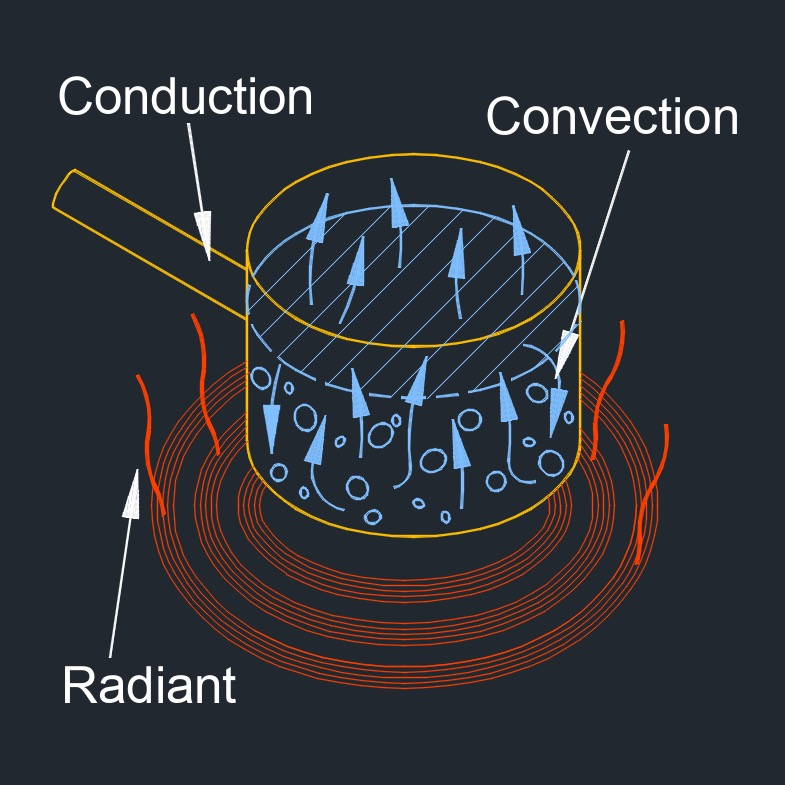# Convective Heat Transfer Coefficient

on . Posted in ThermodynamicsConvective heat transfer coefficient, abbreviated as $$h_c$$, also called film coefficient or film effectiveness, is a porportional constant between heat flux and force from the flow of heat, this also depends on the type of fluid and fluid velocity.

## Convective Heat Transfer Coefficient Formula

$$\large{ h_c = \frac { Q } { \Delta T } }$$
Symbol English Metric
$$\large{ h_c }$$  = heat transfer coefficient  $$\large{\frac{Btu}{hr-ft^2-F}}$$  $$\large{\frac{W}{m^2-K}}$$
$$\large{ Q }$$ = amount of heat transfered area of the surface $$\large{\frac{Btu}{hr}}$$ $$\large{ W }$$
$$\large{ \Delta T }$$ = temperature differential between solid surface and surrounding fluid area $$\large{ F }$$ $$\large{ K }$$